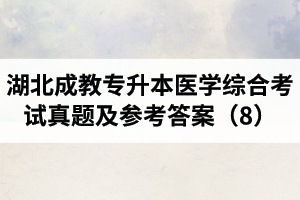﻿ 湖北成教专升本医学综合考试真题及参考答案（8）_湖北成教网

# 湖北成教专升本医学综合考试真题及参考答案（8）

2020-11-18 整编：湖北成教网 阅读：100次

【要学历，上湖北成教网】A.颈椎

B.胸椎

C.腰椎

D.骶椎

E.尾椎

A.肩胛骨关节盂

B.尺骨小头

C.肱骨小头

D.肩胛骨喙突

E.肩胛骨肩峰

A.腹内斜肌腱膜

B.腹外斜肌腱膜

C.腹横肌腱膜

D.腹直肌腱膜

E.腹直肌鞘

A.十二指肠上部

B.十二指肠水平部

C.十二指肠升部

D.十二指肠空肠曲

E.十二指肠降部

A.上颌窦

B.额窦

C.蝶窦

D.筛窦前、中群

E.筛窦后群

A.直肠膀胱陷凹

B.膀胱子宫陷凹

C.直肠子宫陷凹

D.坐骨肛门窝

E.卵巢窝

A.肝肾隐窝

B.肋膈隐窝

C.膀胱子宫陷凹

D.直肠子宫陷凹

E.直肠膀胱陷凹

A.子宫阔韧带

B.子宫圆韧带

C.骶子宫韧带

D.子宫主韧带

E.卵巢悬韧带

A.输卵管漏斗

B.输卵管壶腹

C.输卵管峡

D.输卵管子宫部

E.输卵管壁内部

A.右腋前线与肋弓下缘交点的下方

B.右锁骨中线与肋弓下缘交点的下方

C.剑突的下方

D.剑突右缘与肋弓下缘交点下方

E.右胸骨旁线与肋弓下缘交点下方

A.前列腺部

B.海绵体部

C.尿道球

D.膜部

E.尿道内口

A.左心房

B.右心房

C.左心室

D.右心室

E.左心耳

A.右颈干

B.左颈干

C.肠干

D.右腰干

E.左腰干

A.圣面肠动脉

B.回结肠动脉

C.右结肠动脉

D.中结肠动脉

E.胰十二指肠上动脉

A.迷走神经

B.舌咽神经

C.舌下神经

D.副神经

E.前庭蜗神经

A.一般内脏运动柱

B.特殊躯体感觉柱

C.一般躯体运动柱

D.一般内脏感觉柱

E.特殊内脏运动柱

A.额上回后部

B.额中回后部

C.额下回后部

D.颞上回后部

E.颞下回后部

A.桡神经

B.腋神经

C.尺神经

D.正中神经

E.肌皮神经

A.胸2

B.胸4

C.胸6

D.胸8

E.胸10

A.上睑提肌

B.内直肌

C.下直肌

D.下斜肌

E.上斜肌

A.耳蜗

B.前庭

C.膜半规管

D.咽鼓管

E.蜗管

A.反应

B.反应性

C.兴奋性

D.兴奋

E.抑制

A.物质从高浓度侧向低浓度侧的转运

B.不消耗能量

C.不需要蛋白质的帮助

D.以载体为中介

E.被动转运

A.K+的平衡电位

B.Na+的平衡电位

C.Ca2+的平衡电位

D.Cl-的平衡电位

E.Mg2+的平衡电位

A.8%

B.20%

C.40%

D.60%

E.70%

A.骨髓

B.脾脏

C.心脏

D.肝脏

E.肾脏

A.P波

B.QRS波群

C.T波

D.U波

E.J波

A.大动脉和中动脉

B.小动脉和微动脉

C.毛细血管

D.小静脉和中静脉

E.大静脉

A.心室、心房均收缩

B.主动脉瓣开放

C.房室瓣开放

D.室内压降低

E.心室容积不变

A.物理溶解

B.氨基甲酰血红蛋白

C.碳酸

D.氧合血红蛋白

E.碳酸氢盐

A.潮气量-无效腔气量

B.潮气量×呼吸频率

C.(潮气量-无效腔气量)×呼吸频率

D.无效腔气量×呼吸频率

E.潮气量+无效腔气量

A.唾液

B.胃液

C.胆汁

D.小肠液

E.胰液

A.食管

B.胃

C.小肠

D.结肠

E.直肠

A.肝脏

B.皮肤

C.肺脏

D.肾脏

E.大脑

A.肾小球滤过率/肾血浆流量

B.肾血浆流量/肾血流量

C.肾血流量/肾血浆流量

D.肾小球滤过率/肾血流量

E.肾血流量/心输出量

A.近曲小管

B.髓袢

C.髓袢升支

D.远曲小管

E.集合管

A.明适应

B.瞳孔对光反射

C.近点

D.暗适应

E.视野

A.突触后膜对Na+通透性增大

B.突触后膜对Ca2+、K+通透性增大

C.突触后膜去极化

D.突触后膜超极化

E.突触后膜复极化

A.尖锐刺痛

B.定位不精确

C.对牵拉不敏感

D.对切割敏感

E.对电刺激敏感

A.由丘脑向大脑皮质点对点投射

B.引起特定感觉

C.维持大脑清醒状态

D.所有感觉的上行传导道

E.不接受网状结构上行传人

A.胰岛素

B.肾上腺素

C.甲状腺激素

D.糖皮质激素

E.生长抑素

A.饮食中碘摄入不足

B.食物中缺乏酪氨酸

C.三碘甲腺原氨酸过多

D.四碘甲腺原氨酸过多

E.促甲状腺激素过少

A.心源性

B.肝源性

C.肾源性

D.黏液性

E.营养不良性

A.自发性气胸

B.心力衰竭

C.甲状腺炎

D.支气管炎

E.胸膜炎

A.稽留热

B.弛张热

C.不规则热

D.波状热

E.回归热

A.双手掌平置于肩胛间区

B.双手尺侧缘置于肩胛间区

C.单手指腹置于肩胛间区

D.双手指腹置于肩胛间区

E.双手大鱼际置于肩胛间区

A.肺气肿

B.大叶性肺炎

C.支气管炎

D.胸腔积液

E.气胸

A.心力衰竭

B.二尖瓣狭窄

C.主动脉瓣狭窄

D.高血压

E.房间隔缺损

A.左、右心室收缩不同步

B.左、右心室舒张受限

C.右心回心血量增加

D.右心排血时间延长

E.深吸气末胸腔负压增加

A.半卧位时颈静脉怒张

B.坐位时颈动脉搏动增强

C.坐位时可闻及颈部血管杂音

D.平卧位时可见心尖抬举样搏动

E.平卧位时心底部浊音界明显增宽

A.心包摩擦音

B.心浊音界扩大

C.心尖搏动消失

D.Ewart征

E.毛细血管搏动征

A.短绌脉

B.水冲脉

C.交替脉

D.奇脉

E.重搏脉

A.急性后位阑尾炎

B.急性前位阑尾炎

C.急性高位阑尾炎

D.急性胰腺炎

E.急性肾盂肾炎

A.输尿管结石

B.急性膀胱炎

C.急性肾盂肾炎

D.急性阑尾炎

E.急性肠炎

A.右心衰竭

B.缩窄性心包炎

C.门静脉高压症

D.肾静脉血栓

E.下腔静脉阻塞

A.振水音检查

B.肠鸣音检查

C.鼓音区检查

D.蠕动波检查

E.移动性浊音检查

A.腹膜刺激征阳性

B.夏柯征阳性

C.墨菲征阳性

D.库瓦济埃征阳性

E.肝颈静脉回流征阳性

A.布鲁津斯基征阳性

B.霍夫曼征阳性

C.克匿格征阳性

D.查多克征阳性

E.巴宾斯基征阳性

A.胆红素尿

B.血红蛋白尿

C.血尿

D.脓尿

E.乳糜尿

A.妊娠

B.运动

C.伤寒

D.败血症

E.痈

A.颗粒管型

B.细胞管型

C.蜡样管型

D.脂肪管型

E.透明管型

A.脑脊液检查

B.浆膜腔积液检查

C.血清检查

D.尿液检查

E.粪便检查

A.a1-微球蛋白测定

B.糖化血红蛋白测定

C.血清单胺氧化酶测定

D.血清肌酐测定

E.血氨测定

A.二尖瓣狭窄

B.二尖瓣关闭不全

C.三尖瓣狭窄

D.三尖瓣关闭不全

E.主动脉瓣狭窄

A.低钠血症

B.低钾血症

C.低钙血症

D.高镁血症

E.高铁血症

A.急性前间壁心肌梗死

B.急性广泛前壁心肌梗死

C.急性下壁心肌梗死

D.急性正后壁心肌梗死

E.急性右室心肌梗死

A.仰卧位

B.俯卧位

C.侧卧位

D.半卧位

E.截石位

A.血压低、脉压显著缩小

B.血压低、脉压轻度缩小

C.血压正常、脉压无变化

D.血压正常、脉压缩小

E.血压轻度变低、脉压无变化

A.低钾低氯性碱中毒

B.低钾高氯性碱中毒

C.高钾低氯性碱中毒

D.高钾高氯性碱中毒

E.高钾高氯性酸中毒

A.由β-溶血性链球菌引起

B.属于网状淋巴管炎

C.足癣可为诱发因素

D.好发于下肢及面部

E.蔓延快，常伴有组织坏死

A.口渴严重

B.视觉模糊

C.尿量减少

D.脉搏稍快

E.血压偏低

A.局部隆起

B.有波动感

C.疼痛部位出现可凹性水肿

D.病变部有红、肿、热、痛

E.与正常组织分界清楚，压痛明显

A.20次/分

B.25次/分

C.30次/分

D.35次/分

E.40次/分

A.20～40mmHg

B.50～70mmHg

C.95～100mmHg

D.110～130mmHg

E.140～150mmHg

A.减少肺部并发症

B.减少下肢血栓形成

C.有利于减轻切口疼痛

D.有利于减少尿潴留

E.有利于减轻腹胀

A.可向全血中加入药物

B.可用10%葡萄糖冲洗输血器

C.输血后，血袋应立即扔掉

D.可用生理盐水冲洗输血器

E.疑有溶血反应时，应减慢输血速度

A.维持良好的呼吸功能

B.确保循环功能稳定

C.防治肾衰竭

D.脑复苏

E.用纯氧进行呼吸支持

A.I类切口甲级愈合

B.Ⅱ类切口甲级愈合

C.I类切口乙级愈合

D.Ⅱ类切口乙级愈合

E.I类切口丙级愈合

A.3天

B.1周

C.2周

D.3周

E.5周

A.急性阑尾炎阑尾切除术

B.胃癌根治术

C.嵌顿疝修补术

D.脾破裂的脾切除术

E.消化性溃疡胃大部切除术

A.保持安静

B.防止窒息

C.保证进食

D.减少氧的消耗

E.防止坠床

A.纠正水、电解质及酸碱失衡

B.改善营养状况

C.施行有感染可能的手术，术前使用抗生素

D.手术应在当日尽早进行，缩短术前禁食时间

E.应用胰岛素，使血糖稳定于正常水平

A.呈膨胀性生长，发展较慢

B.有明显包膜与周围组织分界明显

C.不会发生恶变

D.不发生转移

E.极少威胁生命

A.鼻导管吸氧6～8L/min

B.限制输液量和速度

C.应用支气管扩张药

D.进展期呼气终末正压通气

E.不必应用抗生素

A.肋胸膜

B.胸膜顶

C.纵隔胸膜

D.膈胸膜

E.脏胸膜

A.肝胃韧带

B.肝十二指肠韧带

C.胃结肠韧带

D.肝圆韧带

E.肝镰状韧带

A.奇静脉

B.锁骨下静脉

C.头静脉

D.肺静脉

E.颈外静脉

A.铁和蛋白质

B.内因子

C.维生素K

D.维生素B12和叶酸

E.促红细胞生成素

A.盐酸

B.胃蛋白酶原

C.黏液和碳酸氢盐

D.内因子

E.淀粉酶

A.动作电位

B.阈电位

C.局部电位

D.静息电位

E.后电位

A.大量胸腔积液

B.支气管扩张症

C.肺气肿

D.肺不张

E.胸主动脉瘤

A.胸膜肥厚粘连

B.先天性

C.肺不张

D.肺结核

E.肺气肿

A.病理反射

B.牵张反射

C.自主神经反射

D.浅反射

E.深反射

A.呼吸衰竭

B.上消化道出血

C.失血性休克

D.贫血

E.幽门螺杆菌感染

A.限制入水量

B.补充适量液体

C.10%葡萄糖酸钙静脉输入

D.11.2%乳酸钠静脉输入

E.3%高渗盐水静脉输入

A.中心静脉压低、血压低

B.中心静脉压正常、血压低

C.中心静脉压低、血压正常

D.中心静脉压高、血压低

E.中心静脉压高、血压正常

A.输精管壶腹

B.尿道球腺

C.附睾

D.精囊腺

E.前列腺

A.椎动脉

B.胸廓内动脉

C.甲状腺下动脉

D.枕动脉

E.面动脉

A.前核群

B.内侧核群

C.腹前核

D.腹中间核

E.腹后核

A.作用持久

B.作用面广泛

C.定位准确

D.无正反馈

E.反应缓慢

A.是一个密闭的潜在的腔

B.在呼吸时，胸膜腔的容积有变化

C.腔内有少量浆液

D.平静呼吸时，胸膜腔内压始终低于大气压

E.某些生理情况下，胸膜腔内压可以高于大气压

A.滤过膜的通透性和有效滤过面积

B.肾血浆流量

C.肾小球毛细血管血压

D.血浆晶体渗透压

E.肾小球囊内压

A.拉赛格征

B.克匿格征

C.布鲁津斯基征

D.踝阵挛

E.颈强直

A.坐位

B.半卧位

C.仰卧位

D.左侧卧位

E.膝胸位

A.白血病

B.肾结核

C.肝硬化

D.肠梗阻

E.伤寒

A.血钾&lt;3.5mmol/L

B.软瘫

C.心律失常

D.心电图出现U波

E.心脏骤停

A.严重创伤

B.重度烧伤

C.严重感染

D.大手术后

E.腹股沟淋巴结炎

A.微动脉收缩

B.微静脉开放

C.动、静脉短路和直捷通道开放

D.毛细血管前括约肌收缩

E.毛细血管后括约肌开放﻿